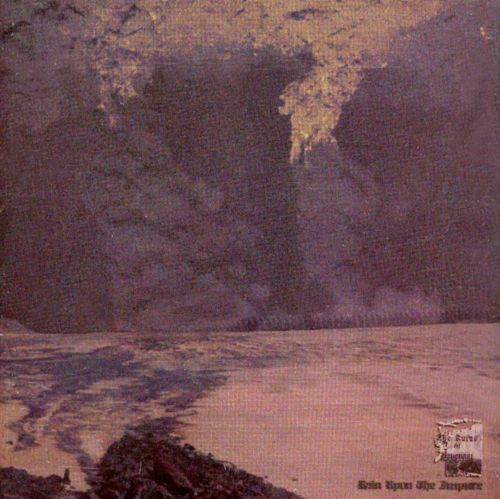# 08.10.2018: One Chapter of Nonfiction

Today's soundtrack is The Ruins of Beverast: Rain Upon the Unjust, an epic atmospheric black metal album.

This afternoon, I'm reading the fifteenth chapter of Larry Gonick's The Cartoon Guide to Physics, "Electric Currents."Alessandro Volta invented "the electric battery in 1794" (p. 128). He found that two rods made of different kinds of metals will show "a difference in potential" (p. 128) when partially submerged in an acid bath. Totally metal.

He found that using a wire to connect the two rods, or terminals, would carry the charge between the terminals; connecting wires from each rod to something else would move the charge to the other thing. This is the essence of a battery: something that converts energy into electricity so that we can use the electricity as a source of power (Paraphrased from this definition).

Another way that we can create a Voltaic cell is to push two nails into a lemon: one copper, one zinc. If we connect wires to each nail and touch the wires to something else, charge is transferred through the wires to the second object. We can even connect the lemons in a series and make even more charge. Real batteries are made up of a bunch of cells in series.

We can create a circuit by setting up our cells and our wires in a loop. The flow of charge that goes around and around a circuit is called the current, which is measured in amperes. Current flows from the positive terminal to the negative terminal.

We need a high voltage to get a high current. George Ohm found the calculation, which we now call Ohm's Law: i = v/r. "Current, , equals voltage, v, divided by resistance, r. The higher the voltage, the more current flows through a given resistance" (p. 131). Factors that contribute to a material's resistance include "the material, the area through which current flows, and the length it must travel" (p. 132). We describe materials through which current flows easily as having low resistivity, and materials through which current has a difficult time flowing as having high resistivity. The higher a temperature of a given material, the more resistive it is.

Superconductors are such materials as "mercury and aluminum" (p. 134) that have zero resistivity when they are at absolute zero. But such materials are very expensive; so for general purposes, we use copper wire, which has a very low resistance at regular temperatures.

So we know about current and resistance. Those are contributing factors that we can ultimately measure in how much power a thing has: how many watts it is. "A watt is one joule per second" (p. 140). We can figure out how much power a thing as with the equation P = Vi, which means "the product of voltage times current is power" (p. 141).#TheCartoonGuidetoPhysics